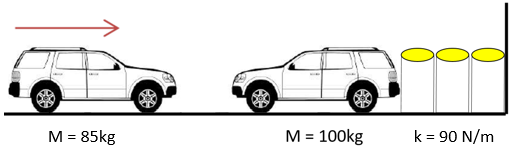## Water Barrel Collision

In the below figure, a 85 kg car collides with a 100 kg car that is at rest next to some water barrels you typically find on the highway. The barrels act as a spring between the vehicle and fixed wall to reduce impact. If the barrels have a spring constant of 90 kN/m, and the larger car is observed to have moved 6 m/s immediately after impact, what is the max acceleration of the 100 kg car after impact?Hint
The spring’s potential energy:
$$U=\frac{kx^{2}}{2}$$$where $$k$$ is the spring constant, and $$x$$ is the compressed distance. Hint 2 The larger car's kinetic energy: $$KE=\frac{1}{2}mv^2$$$
where $$m$$ is the mass, and $$v$$ is the velocity.
The spring's potential energy:
$$U=\frac{kx^{2}}{2}=\frac{90N(x)^2}{2\cdot m}=45(x)^2N/m$$$where $$k$$ is the spring constant, and $$x$$ is the compressed distance. The larger car's kinetic energy: $$KE=\frac{1}{2}mv^2=\frac{1}{2}(100kg)(6m/s)^2=1,800\:N\cdot m$$$
where $$m$$ is the mass, and $$v$$ is the velocity. Because energy is conserved, $$U=KE$$
$$1,800N\cdot m=45(x)^2\frac{N}{m}$$$$$40m^2=(x)^2$$$
$$x=\sqrt{40m^2}=6.325\:m$$$The spring compressed 6.325 m when the larger car was observed travelling at 6 m/s. At max compression, the spring force is given by: $$F=kx=mass\times acceleration$$$
$$(90N/m)(6.325m)=(100kg)\times a$$$$$a=\frac{569.2N}{100kg}=5.69\:\frac{m}{s^2}$$$
$$5.69\:\frac{m}{s^2}$$\$